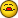# Hide Zero Values

P

#### PaulOakley

Hello,

I need to run a macro to hide any rows that contain a zero value in a
certain column number. e.g hide all rows that contain a zero value in
column B?

Is this possible do you think?

Thanks in advance

Paul

Ad

B

#### Bob Phillips

Sub Test()
Dim iLastRow As Long
Dim i As Long
Dim rng As rng

iLastRow = Cells(Rows.Count, "B").End(xlUp).Row
For i = 1 To iLastRow
If Cells(i, "B").Value = 0 Then
If rng Is Nothing Then
Set rng = Rows(i)
Else
Set rng = Union(rng, Rows(i))
End If
End If
Next i

If Not rng Is Nothing Then rng.Hidden = True

End Sub

--
HTH

Bob Phillips

(remove nothere from email address if mailing direct)

A

#### Ardus Petus

Typo:

Dim rng as Range

HTH
--
AP

Bob Phillips said:
Sub Test()
Dim iLastRow As Long
Dim i As Long
Dim rng As rng

iLastRow = Cells(Rows.Count, "B").End(xlUp).Row
For i = 1 To iLastRow
If Cells(i, "B").Value = 0 Then
If rng Is Nothing Then
Set rng = Rows(i)
Else
Set rng = Union(rng, Rows(i))
End If
End If
Next i

If Not rng Is Nothing Then rng.Hidden = True

End Sub

--
HTH

Bob Phillips

(remove nothere from email address if mailing direct)

in message news[email protected]

P

#### PaulOakley

Seems to be coming back with error msg of "user defined ype no
defined"

Dim rng As rng- this part is being highlighted, is this right, o
should i be entering data her

P

#### PaulOakley

If Not rng Is Nothing Then rng.Hidden = True

This is now showing as a debug error.. I know very little VBA, so don
seem to be able to change the error..

Thanks in advanc

B

#### Bob Phillips

Thanks mate.

--
HTH

Bob Phillips

(remove nothere from email address if mailing direct)

Ad

B

#### Bob Phillips

Paul,

Ardus pointed out a typo, and I need a bit more property

Sub Test()
Dim iLastRow As Long
Dim i As Long
Dim rng As Range

iLastRow = Cells(Rows.Count, "B").End(xlUp).Row
For i = 1 To iLastRow
If Cells(i, "B").Value = 0 Then
If rng Is Nothing Then
Set rng = Rows(i)
Else
Set rng = Union(rng, Rows(i))
End If
End If
Next i

If Not rng Is Nothing Then rng.EntireRow.Hidden = True

End Sub

--
HTH

Bob Phillips

(remove nothere from email address if mailing direct)

## Ask a Question

Want to reply to this thread or ask your own question?

You'll need to choose a username for the site, which only take a couple of moments. After that, you can post your question and our members will help you out.